Back to: Chromatic Polynomial
##### Case 2.1: Calculation of $$P_{2.1^{\circ}}(k)$$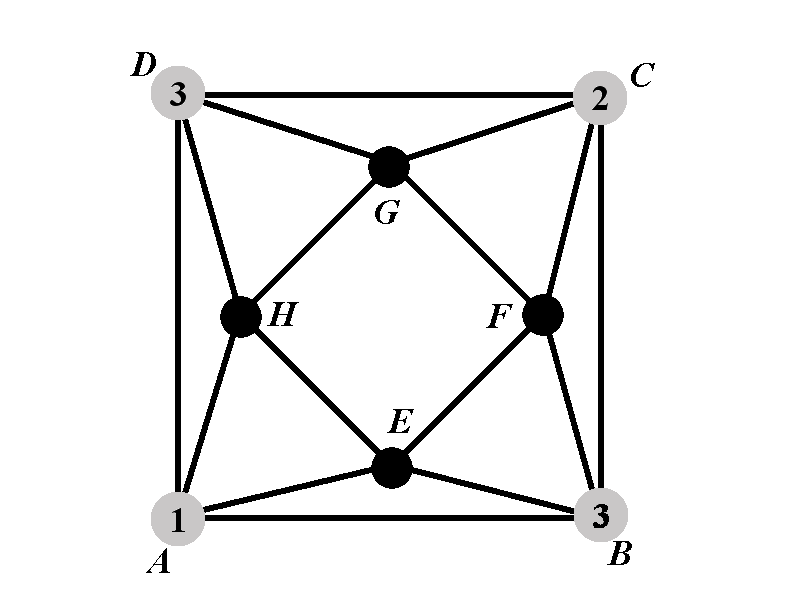Observe that none of the vertices $$E$$ and $$H$$ can have color $$1$$ or color $$3$$. We will consider two sub-cases: Sub-case 2.1.1 is when one of the vertices $$E$$ and $$H$$ has the color $$2$$. The sub-case 2.1.1 is when none of $$E$$ and $$H$$ has a color from $$\{1,2,3\}$$.

In the case 2.1.1 the number of colorings in which $$E$$ has the color $$2$$ is equal to the number of colorings in which $$H$$ has the color $$2$$. If the vertex $$E$$ has the color $$2$$ then there are $$k-3$$ choices for the color of $$H$$. Let us denote by $$P_{2.1.1^{\circ}}(k)$$ the number of proper colorings of $$\Gamma$$ in which $$A$$, $$B$$, $$C$$, $$D$$, $$E$$, and $$H$$ have the colors $$1$$, $$3$$, $$2$$, $$3$$, $$2$$, and $$4$$. The total number of colorings in the case 2.1.1 is $$2\cdot (k-3)\cdot P_{2.1.1^{\circ}}(k)$$.

In the case 2.1.2 the vertices $$E$$ and $$H$$ cannot have any of the colors from $$\{1,2,3\}$$. In addition, the vertices $$E$$ and $$H$$ must be of mutually different colors. There are $$k-3$$ choices for the color of $$E$$ and for each of those choices there are $$k-4$$ available colors for $$H$$. Let us denote by $$P_{2.1.2^{\circ}}(k)$$ the number of proper colorings of $$\Gamma$$ in which $$A$$, $$B$$, $$C$$, $$D$$, $$E$$, and $$H$$ have the colors $$1$$, $$3$$, $$2$$, $$3$$, $$4$$, and $$5$$, respectively. The total number of colorings in the case 2.1.2 is $$(k-3)(k-4)P_{2.1.2^{\circ}}(k)$$.

Therefore the polynomial $$P_{2.1^{\circ}}(k)$$ satisfies $P_{2.1^{\circ}}=2(k-3)P_{2.1.1^{\circ}}(k)+(k-3)(k-4)P_{2.1.2^{\circ}}(k).$

##### Case 2.1.1: Calculation of $$P_{2.1.1^{\circ}}(k)$$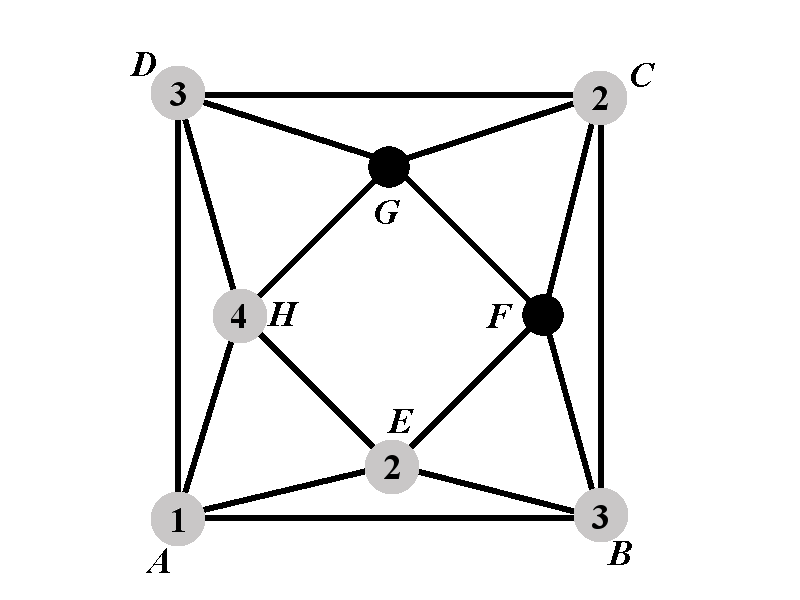The vertex $$G$$ cannot have any of the colors $$2$$, $$3$$, and $$4$$. There are $$k-3$$ colors available for $$G$$. For any of those $$k-3$$ choices there are exactly $$k-3$$ colors available for $$F$$ (the color $$4$$ is back as an option for $$F$$, but the color that $$G$$ took is out). Therefore $P_{2.1.1^{\circ}}(k)=(k-3)^2.$

##### Case 2.1.2: Calculation of $$P_{2.1.2^{\circ}}(k)$$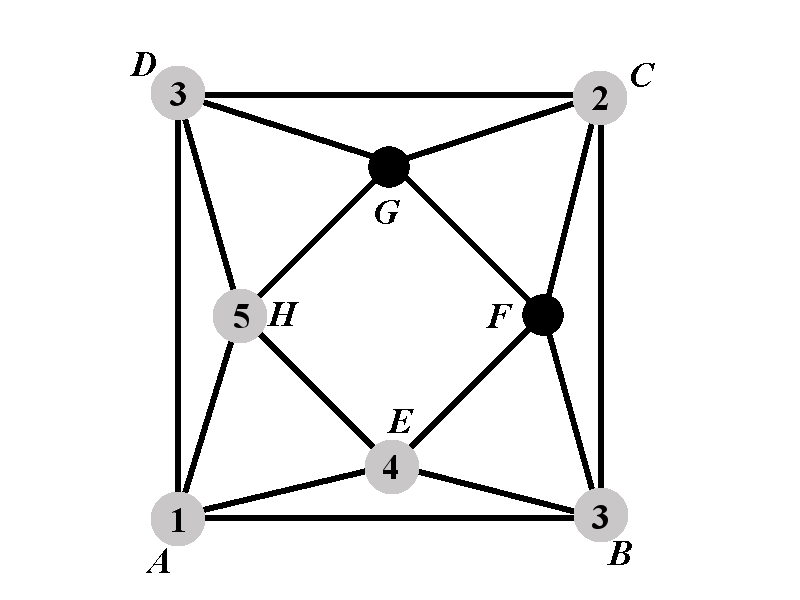The case 2.1.2 further brakes into two sub-cases. Let us observe the vertex $$F$$. The vertex $$F$$ cannot have any of the colors from the set $$\{2,3,4\}$$. We will consider the sub-case 2.1.2.1 in which the vertex $$F$$ has the color 5, and the sub-case 2.1.2.2 in which the vertex $$F$$ has a color outside of the set $$\{2,3,4,5\}$$. In the sub-case 2.1.2.2 there are $$k-4$$ possibilities for $$F$$.

Denote by $$P_{2.1.2.1^{\circ}}(k)$$ the number of proper colorings of $$\Gamma$$ such that the vertices $$A$$, $$B$$, $$C$$, $$D$$, $$E$$, $$F$$, and $$H$$ have the colors $$1$$, $$3$$, $$2$$, $$3$$, $$4$$, $$5$$, and $$5$$, respectively. Denote by $$P_{2.1.2.2^{\circ}}(k)$$ the number of proper colorings of $$\Gamma$$ such that the vertices $$A$$, $$B$$, $$C$$, $$D$$, $$E$$, $$F$$, and $$H$$ have the colors $$1$$, $$3$$, $$2$$, $$3$$, $$4$$, $$1$$, and $$5$$, respectively.

The number $$P_{2.1.2^{\circ}}(k)$$ satisfies $P_{2.1.2^{\circ}}(k)=P_{2.1.2.1^{\circ}}(k)+(k-4)P_{2.1.2.2^{\circ}}(k).$

##### Case 2.1.2.1: Calculation of $$P_{2.1.2.1^{\circ}}(k)$$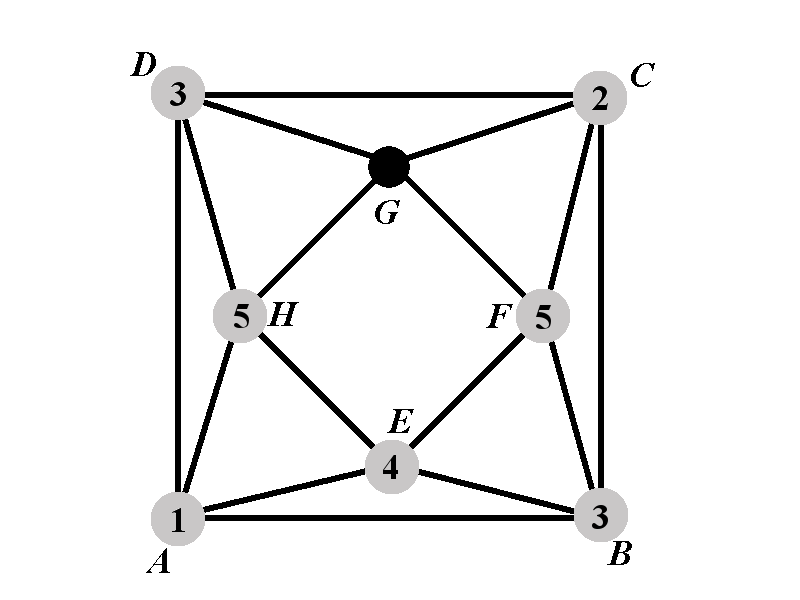It remains to count the number of ways to color the vertex $$G$$ that would complete the proper coloring of $$\Gamma$$. The vertex $$G$$ cannot have any of the colors from the set $$\{2,3,5\}$$. Therefore there are $$k-3$$ available colors for $$G$$. Hence $P_{2.1.2.1^{\circ}}(k)=k-3.$

##### Case 2.1.2.2: Calculation of $$P_{2.1.2.2^{\circ}}(k)$$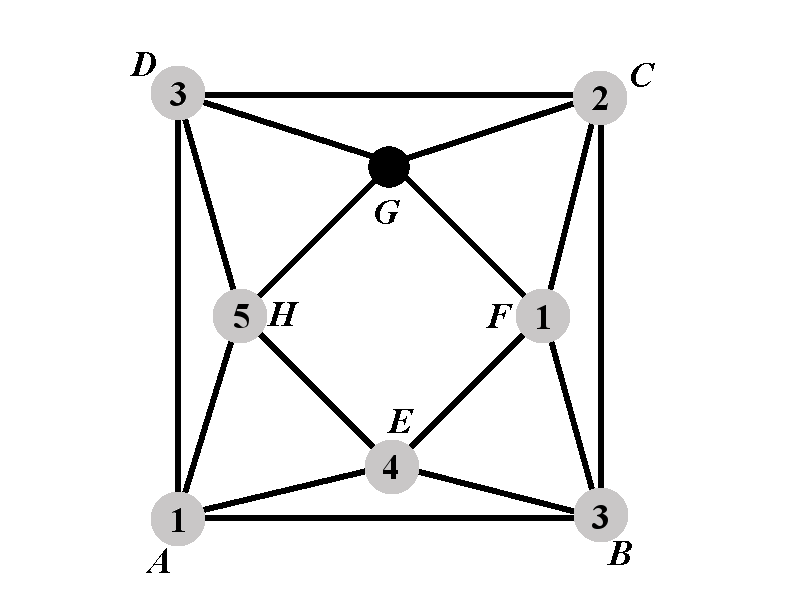The vertex $$G$$ cannot have any of the colors from the set $$\{1,2,3,5\}$$. Therefore there are $$k-4$$ available colors for $$G$$ and $P_{2.1.2.2^{\circ}}(k)=k-4.$

##### Final Calculation of $$P_{2.1^{\circ}}(k)$$

We now have all the ingredients to complete the calculation of $$P_{2.1^{\circ}}(k)$$. \begin{eqnarray*}P_{2.1^{\circ}}(k)&=&2(k-3)P_{2.1.1^{\circ}}(k)+(k-3)(k-4)P_{2.1.2^{\circ}}(k)\newline &=&2(k-3)\cdot (k-3)^2+(k-3)(k-4)\left(P_{2.1.2.1^{\circ}}(k)+(k-4)P_{2.1.2.2^{\circ}}(k)\right)\newline &=&2(k-3)^3+(k-3)(k-4)\left(k-3+(k-4)\cdot(k-4)\right)\newline &=&(k-3)\left[2(k-3)^2+(k-3)(k-4)+(k-4)^3\right]\newline &=&(k-3)\left(k^3-9k^2+29k-34\right). \end{eqnarray*}